xSurface energyEncyclopedia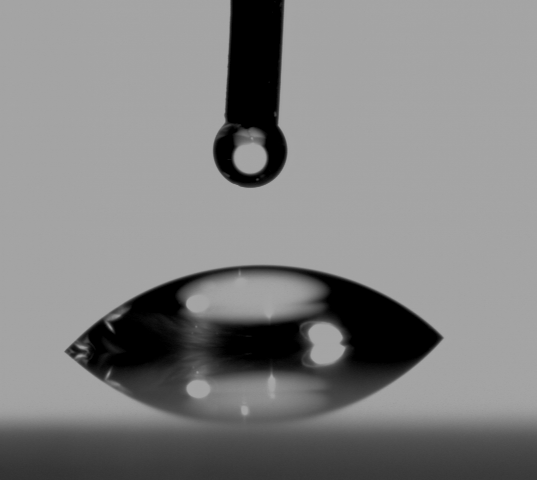Surface energy quantifies the disruption of intermolecular bonds that occur when a surface is created. In the physics
Physics
Physics is a natural science that involves the study of matter and its motion through spacetime, along with related concepts such as energy and force. More broadly, it is the general analysis of nature, conducted in order to understand how the universe behaves.Physics is one of the oldest academic...

of solid
Solid
Solid is one of the three classical states of matter . It is characterized by structural rigidity and resistance to changes of shape or volume. Unlike a liquid, a solid object does not flow to take on the shape of its container, nor does it expand to fill the entire volume available to it like a...

s, surfaces must be intrinsically less energetically favorable than the bulk of a material, otherwise there would be a driving force for surfaces to be created, removing the bulk of the material (see sublimation). The surface energy may therefore be defined as the excess energy at the surface of a material compared to the bulk.

For a liquid, the surface tension
Surface tension
Surface tension is a property of the surface of a liquid that allows it to resist an external force. It is revealed, for example, in floating of some objects on the surface of water, even though they are denser than water, and in the ability of some insects to run on the water surface...

(force per unit length) and the surface energy density are identical. Water has a surface energy density of 0.072 J/m2 and a surface tension of 0.072 N/m.

Cutting a solid body into pieces disrupts its bonds, and therefore consumes energy. If the cutting is done reversibly (see reversible
Reversibility
Reversibility can refer to:* Reversible dynamics, a mathematical dynamical system, or physical laws of motion, for which time-reversed dynamics are well defined* Reversible diffusion, an example of a reversible stochastic process...

), then conservation of energy
Conservation of energy
The nineteenth century law of conservation of energy is a law of physics. It states that the total amount of energy in an isolated system remains constant over time. The total energy is said to be conserved over time...

means that the energy consumed by the cutting process will be equal to the energy inherent in the two new surfaces created. The unit surface energy of a material would therefore be half of its energy of cohesion
Cohesion
Cohesion may refer to:* Cohesion , the intermolecular attraction between like-molecules* Cohesion , a measure of how well the lines of source code within a module work together...

, all other things being equal; in practice, this is true only for a surface freshly prepared in vacuum. Surfaces often change their form away from the simple "cleaved bond" model just implied above. They are found to be highly dynamic regions, which readily rearrange or react, so that energy is often reduced by such processes as passivation
Passivation
Passivation is the process of making a material "passive", and thus less reactive with surrounding air, water, or other gases or liquids. The goal is to inhibit corrosion, whether for structural or cosmetic reasons. Passivation of metals is usually achieved by the deposition of a layer of oxide...

Adsorption is the adhesion of atoms, ions, biomolecules or molecules of gas, liquid, or dissolved solids to a surface. This process creates a film of the adsorbate on the surface of the adsorbent. It differs from absorption, in which a fluid permeates or is dissolved by a liquid or solid...

.

## Measuring the surface energy of a liquid

As first described by Thomas Young
Thomas Young (scientist)
Thomas Young was an English polymath. He is famous for having partly deciphered Egyptian hieroglyphics before Jean-François Champollion eventually expanded on his work...

in 1805 in the Philosophical Transactions of the Royal Society of London, it is the interaction between the forces of cohesion and the forces of adhesion
Adhesion is any attraction process between dissimilar molecular species that can potentially bring them in close contact. By contrast, cohesion takes place between similar molecules....

which determines whether or not wetting
Wetting
Wetting is the ability of a liquid to maintain contact with a solid surface, resulting from intermolecular interactions when the two are brought together. The degree of wetting is determined by a force balance between adhesive and cohesive forces.Wetting is important in the bonding or adherence of...

, the spreading of a liquid over a surface, occurs. If complete wetting does not occur, then a bead of liquid will form, with a contact angle
Contact angle
The contact angle is the angle at which a liquid/vapor interface meets a solid surface. The contact angle is specific for any given system and is determined by the interactions across the three interfaces. Most often the concept is illustrated with a small liquid droplet resting on a flat...

which is a function of the surface energies of the system.
Surface energy is most commonly quantified using a contact angle goniometer
Goniometer
A goniometer is an instrument that either measures an angle or allows an object to be rotated to a precise angular position. The term goniometry is derived from two Greek words, gōnia, meaning angle, and metron, meaning measure....

and a number of different methods.

### Young's equation

Young
Thomas Young (scientist)
Thomas Young was an English polymath. He is famous for having partly deciphered Egyptian hieroglyphics before Jean-François Champollion eventually expanded on his work...

established the well-regarded Young's Equation which defines the balances of forces caused by a wet drop on a dry surface. If the surface is hydrophobic
Hydrophobe
In chemistry, hydrophobicity is the physical property of a molecule that is repelled from a mass of water....

then the contact angle of a drop of water will be larger. Hydrophilicity
Hydrophile
A hydrophile, from the Greek "water" and φιλια "love," is a molecule or other molecular entity that is attracted to, and tends to be dissolved by water. A hydrophilic molecule or portion of a molecule is one that has a tendency to interact with or be dissolved by, water and other polar substances...

is indicated by smaller contact angles and higher surface energy. (Water has rather high surface energy by nature; it is polar
Chemical polarity
In chemistry, polarity refers to a separation of electric charge leading to a molecule or its chemical groups having an electric dipole or multipole moment. Polar molecules interact through dipole–dipole intermolecular forces and hydrogen bonds. Molecular polarity is dependent on the difference in...

and forms hydrogen bond
Hydrogen bond
A hydrogen bond is the attractive interaction of a hydrogen atom with an electronegative atom, such as nitrogen, oxygen or fluorine, that comes from another molecule or chemical group. The hydrogen must be covalently bonded to another electronegative atom to create the bond...

s).
The Young equation gives the following relation,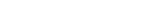where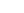,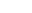, andare the interfacial tensions between the solid and the liquid, the liquid and the vapor, and the solid and the vapor, respectively. The equilibrium
Hydrostatic equilibrium
Hydrostatic equilibrium or hydrostatic balance is the condition in fluid mechanics where a volume of a fluid is at rest or at constant velocity. This occurs when compression due to gravity is balanced by a pressure gradient force...

contact angle that the drop makes with the surface is denoted by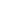. To derive the Young equation, normally the interfacial tensions are described as forces per unit length and from the one-dimensional force balance along theaxis Young equation is obtained.

The Young equation assumes a perfectly flat surface, and in many cases surface roughness and impurities cause a deviation in the equilibrium contact angle from the contact angle predicted by Young's equation. Even in a perfectly smooth surface a drop will assume a wide spectrum of contact angles ranging from the so called advancing contact angle,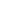, to the so called receding contact angle,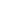. The equilibrium contact angle () can be calculated fromandas was shown by Tadmor as,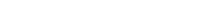where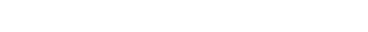In the case of "dry wetting", one can use the Young-Dupré equation
Wetting
Wetting is the ability of a liquid to maintain contact with a solid surface, resulting from intermolecular interactions when the two are brought together. The degree of wetting is determined by a force balance between adhesive and cohesive forces.Wetting is important in the bonding or adherence of...

which is expressed by the work of adhesion
Adhesion is any attraction process between dissimilar molecular species that can potentially bring them in close contact. By contrast, cohesion takes place between similar molecules....

. This method accounts for the surface pressure of the liquid's vapor which can be significant. Pierre-Gilles de Gennes
Pierre-Gilles de Gennes
Pierre-Gilles de Gennes was a French physicist and the Nobel Prize laureate in physics in 1991.-Biography:...

, a Nobel Prize Laureate in Physics, describes wet and dry wetting and how the difference between the two relate to whether or not the vapor is saturated.

## Measuring the surface energy of a solid

The surface energy of a liquid may be measured by stretching a liquid membrane
Artificial membrane
An artificial membrane, or synthetic membrane, is a synthetically created membrane which is usually intended for separation purposes in laboratory or in industry. Synthetic membranes have been successfully used for small and large-scale industrial processes since the middle of twentieth century. A...

(which increases the surface area and hence the surface energy density). In that case, in order to increase the surface area of a mass of liquid by an amount, δA, a quantity of work, γδA, is needed (where γ is the surface energy density of the liquid). However, such a method cannot be used to measure the surface energy of a solid because stretching of a solid membrane induces elastic energy in the bulk in addition to increasing the surface energy.

The surface energy of a solid is usually measured at high temperatures. At such temperatures the solid creeps
Creep (deformation)
In materials science, creep is the tendency of a solid material to slowly move or deform permanently under the influence of stresses. It occurs as a result of long term exposure to high levels of stress that are below the yield strength of the material....

and even though the surface area changes, the volume remains approximately constant. If γ is the surface energy density of a cylindrical rod of radiusand length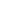at high temperature and a constant uniaxial tension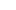, then at equilibrium, the variation
Variation
- Physics :* Magnetic variation, difference between magnetic north and true north, measured as an angle* Variation , any perturbation of the mean motion or orbit of a planet or satellite, particularly of the moon- Mathematics :* Bounded variation...

of the total Gibbs free energy
Gibbs free energy
In thermodynamics, the Gibbs free energy is a thermodynamic potential that measures the "useful" or process-initiating work obtainable from a thermodynamic system at a constant temperature and pressure...

vanishes and we have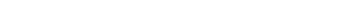where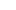is the Gibbs free energy and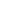is the surface area of the rod: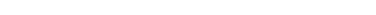Also, since the volume (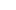) of the rod remains constant, the variation (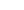) of the volume is zero, i.e.,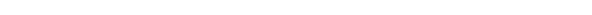Therefore, the surface energy density can be expressed as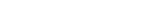The surface energy density of the solid can be computed by measuring,, andat equilibrium.

## Calculating the Surface Energy of a Deformed Solid

In the deformation of solids, surface energy can be treated as the "energy required to create one unit of surface area", and is a function of the difference between the total energies of the system before and after the deformation: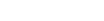.

Calculation of surface energy from first principles is an alternative approach to measurement. Surface energy is estimated from the following variables: width of the d-band, the number of valence d-electrons, and the coordination number
Coordination number
In chemistry and crystallography, the coordination number of a central atom in a molecule or crystal is the number of its nearest neighbours. This number is determined somewhat differently for molecules and for crystals....

of atoms at the surface and in the bulk of the solid.

• Contact angle
Contact angle
The contact angle is the angle at which a liquid/vapor interface meets a solid surface. The contact angle is specific for any given system and is determined by the interactions across the three interfaces. Most often the concept is illustrated with a small liquid droplet resting on a flat...

• Surface tension
Surface tension
Surface tension is a property of the surface of a liquid that allows it to resist an external force. It is revealed, for example, in floating of some objects on the surface of water, even though they are denser than water, and in the ability of some insects to run on the water surface...

• Sessile drop technique
• Capillary surface
Capillary surface
In fluid mechanics and mathematics, a capillary surface is a surface that represents the interface between two different fluids. As a consequence of being a surface, a capillary surface has no thickness in slight contrast with most real fluid interfaces....

• Wulff Construction
Wulff Construction
The Wulff construction is a method for determining the equilibrium shape of a droplet or crystal of fixed volume inside a separate phase...